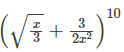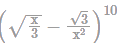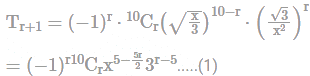The coefficient of the term independent of x...

### Related TestThe coefficient of the term independent of x in the expansion ofisKunaal SatijaThe (r+1) th term in the expansion ofis given byFor term to be independent of x,
5 - (5r/2) = 0
⇒ r = 2
Substituting the values in (1) we get:
T3 = (−1)210C23−3= 10(9)/2(27)
= 5/3
Hence, the correct answer is 5/3.View courses related to this question Explore CAT courses
 Explore CAT coursesView courses related to this question1 Crore+ students have signed up on EduRev. Have you?

• ### Two series of numbers are formed such that the first series is an arithmeti... more(Scan QR code)